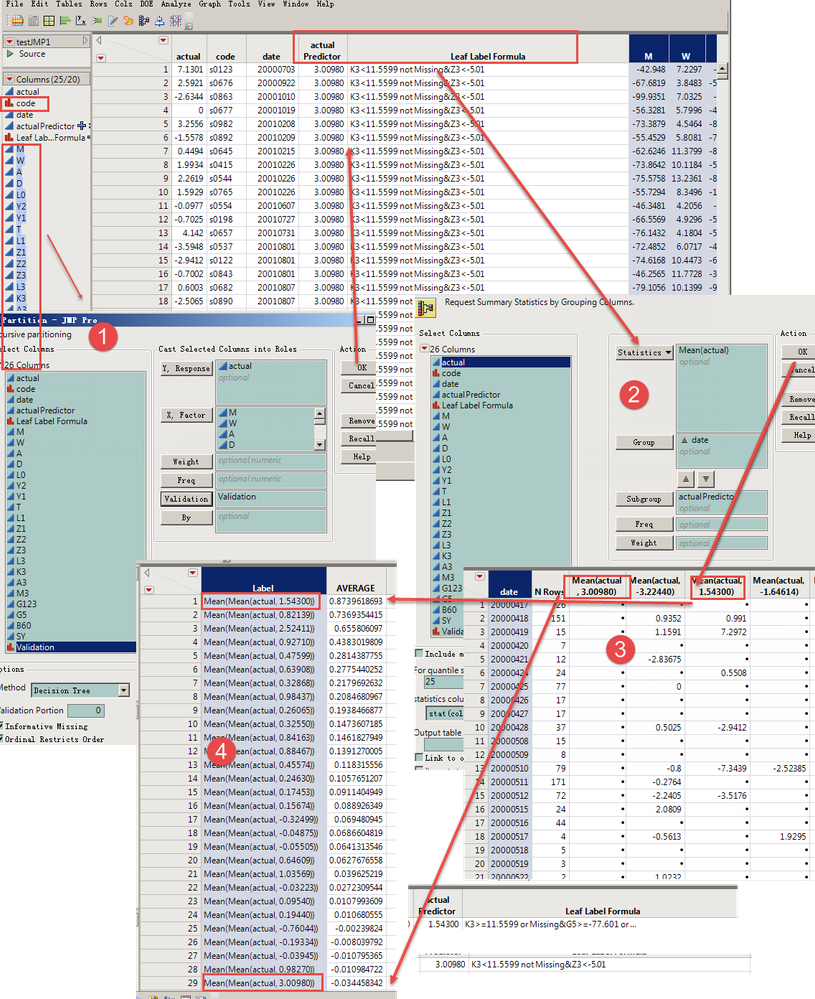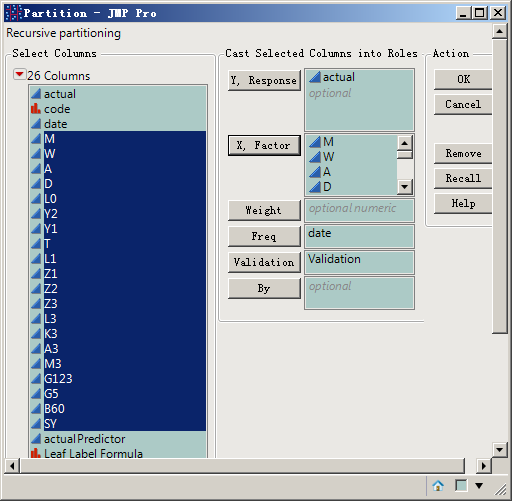Choose Language Hide Translation Bar
Highlighted

## How to control the operation process of "Partition" module in JMP "decision tree" according to the specified "date" condition in actual data?

See attachment and screenshot for specific data and operation steps.My purpose is to use the segmentation module of JMP to calculate the prediction formula. Firstly, the prediction formula is processed by "date", and only one average value is obtained for the same date. Then, the prediction formula is used to average the unique average value of different dates to calculate the prediction value of this formula.
Thanks!

16 REPLIES 16
Highlighted

## Re: How to control the operation process of "Partition" module in JMP "decision tree" according to the specified "date" condition in actual data?

According to each "code" object in the graph file, different index data (X factor) will be generated on different "date", and "actual" a will be generated(Y Response).

Using the "Partition" module of JMP, taking "actual" as the Y Response and "M-BY" as the X factor, and using "training, verification and test" to find out the X factor that is highly correlated with "actual".

And use the "Partition" function to save the predictor value and Leaf label formula.
In this way, JMP can generate better prediction value and Leaf label formula(Setting: The "actual" larger the value, is the better).

But many times the Leaf label formula with a high predictor value will not work best when tested:
I have tested A lot of such data and found that JMP only averages all "actual" that conform to this Leaf label formula without further classification of the "date" factor.

Highlighted

## Re: How to control the operation process of "Partition" module in JMP "decision tree" according to the specified "date" condition in actual data?

For example, if this Leaf label formula in A certain "date", there are many code's "actual" is very large, but in other "date" in line with this Leaf label formula "code" is not many, and "actual" is not good, but according to JMP now is to carry out all the "code" of average calculation, this predictor value is also good

But if according to this Leaf label formula, different "code" of the same "date" to the "date" of the average calculation, which makes this Leaf label formula makes an "actual" each "date", the last in A different "date" to indicate the formula of the average forecast, one way to check, after JMP get better forecast formula of the check result often is not good.
(I would like to make a note here: In order to verify whether JMP already has this feature, I also tried to use the "date" factor as a "freq" option button parameter, but the effect did not meet my requirements.)Highlighted

## Re: How to control the operation process of "Partition" module in JMP "decision tree" according to the specified "date" condition in actual data?

I found my description verbose and didn't know if I had made the problem clear.Please give me advice and help from an experienced expert.Thank you very much!

Highlighted

## Re: JMP is Not a Spreadsheet

I've been using JMP as a quantification tool.

The one I use the most is its "decision tree"-"Partition" module.

In the process of using JMP, I also found that the "decision tree"-"Partition" function of JMP needs to be improved, but I don't know how to feedback my own ideas to JMP company?Make improvements to related functions.

Thanks!

Highlighted

## Re: JMP is Not a Spreadsheet

You can submit suggestions and requests here.

Learn it once, use it forever!
Highlighted

## Re: JMP is Not a Spreadsheet

Thank markbailey for giving me the opportunity to bring this up.

According to each "code" object in the graph file, different index data (X factor) will be generated on different "date", and "actual" a will be generated(Y Response).

Using the "Partition" module of JMP, taking "actual" as the Y Response and "M-BY" as the X factor, and using "training, verification and test" to find out the X factor that is highly correlated with "actual".

And use the "Partition" function to save the predictor value and Leaf label formula.
In this way, JMP can generate better prediction value and Leaf label formula(Setting: The "actual" larger the value, is the better).Highlighted

## Re: JMP is Not a Spreadsheet

But many times the Leaf label formula with a high predictor value will not work best when tested:
I have tested A lot of such data and found that JMP only averages all "actual" that conform to this Leaf label formula without further classification of the "date" factor.

For example, if this Leaf label formula in A certain "date", there are many code's "actual" is very large, but in other "date" in line with this Leaf label formula "code" is not many, and "actual" is not good, but according to JMP now is to carry out all the "code" of average calculation, this predictor value is also good.

• Take the result of step 4 in the figure.

Highlighted

## Re: JMP is Not a Spreadsheet

But if according to this Leaf label formula, different "code" of the same "date" to the "date" of the average calculation, which makes this Leaf label formula makes an "actual" each "date", the last in A different "date" to indicate the formula of the average forecast, one way to check, after JMP get better forecast formula of the check result often is not good.
(I would like to make a note here: In order to verify whether JMP already has this feature, I also tried to use the "date" factor as a "freq" option button parameter, but the effect did not meet my requirements.)Highlighted

## Re: JMP is Not a Spreadsheet

I found my description verbose and didn't know if I had made the problem clear.
My purpose is to use the segmentation module of JMP to calculate the prediction formula. Firstly, the prediction formula is processed by "date", and only one average value is obtained for the same date. Then, the prediction formula is used to average the unique average value of different dates to calculate the prediction value of this formula.
Thanks!

Article Labels

There are no labels assigned to this post.